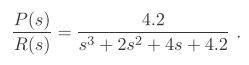# Calculating the transfer function from Laplace Transforms

Lolsauce

## Homework Statement

A Control system for a spacecraft platform is governed by the following equations:

d²p/dt² + 2 dp/dt + 4p = θ
v1 = r - p
dθ/dt = .6*v2
v2 = 7*v1

All the variavles v1, v2, r, p, θ are functions in the time domain

Determine the system transfer function P(s)/R(s)

## Homework Equations

d²x/dt² -> Laplace -> s² * X(s)
dx/dt -> Laplace -> s * X(s)
x(t) -> Laplace -> X(s)

## The Attempt at a Solution

So first I took the Laplace of all the given equations:

d²p/dt² + 2 dp/dt + 4p = θ -> s²*P(s) +2s*P(s) + 4*P(s) = θ(s) )
dθ/dt = .6*v2 -> θ(s) = .6*V2(s)

v1 = r - p -> V1(s) = R(s) - P(s)
v2 = 7*v1 -> V2(s) = 7*V1(s)

I now have four equations I can substitute:
1. s²*P(s) +2s*P(s) + 4*P(s) = θ(s)
2. θ(s) = .6*V2(s)
3. V1(s) = R(s) - P(s)
4. V2(s) = 7*V1(s)

I substitute Eq 3 into Eq 4 and I obtain V2(s) = 7*R(s) - 7*P(s)
then I substitute my new V2(s) equation into Eq 2, which gives me

θ(s) = 4.2*R(s) - 4.2*P(s)

Which I then substitute into Eq 1.

I get a Transfer Function

P(s)/R(s) = 4.2/(s³ + 2s² + 8.2s)

I think I did all my calculations right, but the solution in the book is this:Can somebody tell me what I did wrong?

Last edited:

## Answers and Replies

Homework Helper
Gold Member
dθ/dt = .6*v2 -> θ(s) = .6*V2(s)

You'll want to double check this part